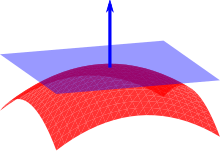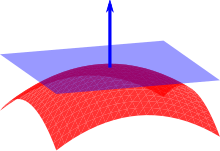# Explanation of Surface Normal?

I know when you raycast, 4 pieces of information are returned, one being the surface normal. Im just wondering what a surface normal is and what I can do with it. I’ve seen stuff on the wiki about reflecting rays using surface normals and I’ve also seen other scripts use them for different things, but I have no idea what it is, what it’s for, or what to do with it.

8 Likes

I looked around the forums and found another similar forum post that was made back in 2015 that gives an explanation of it. I hope it helps:
https://devforum.roblox.com/t/surface-normal-why-vector3/12654

2 Likes

On ROBLOX, the Surface Normal returned by the Raycast function is essentially just a Vector that is perpendicular to the tangential surface which the Raycast has hit.

Here is a pretty good visualization of it from Wikipedia:11 Likes

Does this mean that we can then create another ray which is essentially a reflection of the original ray?

2 Likes

Still a bit confused, isn’t the surface normal just a point? Like, would there be a significant difference between a surface normal of (3,4,3) and (3,7,3)?

2 Likes

## What is it?

A surface normal is a vector.

The normal of a surface is a line which points directly outwards, perfectly flat from the surface. It is considered to be an indication of what direction a point on a surface is facing.

So, the surface normal of a flat surface pointing toward the sky would be:

``````Vector3(0, 1, 0)
``````

It’s also important to note that the vector is usually normalised, so the magnitude is set to 1.

## What it’s for

Surface normals are used by the lighting engine to calculate shine. The more similar the surface normal of a surface and the vector to the light emitter are, the more shine is applied. Quick note: I’m not sure if that is exactly what Roblox’s engine does, but it’s how I’ve seen shine calculated in every lighting engine ever, so I guess we can assume it does.

This is the only area which I’ve ever used it. I’ve oversimplified the exact algorithm a bit, so that it doesn’t fill the entire page, but that’s the basics, and it’s a very common use of the surface normal.

## What to do with it

I’ve seen it used for a lot of things, including calculating the bounce of an object, right up to wall bang in an FPS game.

They’re multipurpose, and, with a bit of math, you can use it to do some very cool simulations.

5 Likes

The surface normal is a vector, which can be imagined as a description of an arrow in 3D space.
In the example given by @jody7777, the arrow is a representation of the vector that is perpendicular (aka normal aka at a 90 degree angle to) the surface at that point.A Vector3 is described as such because it is a vector with 3 components, normally (x, y, z). Now let’s say that the length of the arrow in the above image is 5 units. It is shown to be pointing straight up, in the positive-y direction (if it was pointing straight down, it would be pointing in the negative-y direction).

We can describe this arrow with the vector (0, 5, 0) - it has no unit in the x or z directions, as it is not pointing forwards, backwards, or side-to-side- it is only pointing upwards. Again, if it was pointing straight down we could describe it as (0, -5, 0).

Yes, there would! (3,4,3) can describe an arrow pointing 3 units in the x direction, 4 units in the y direction, and 3 units in the z direction. However (3,7,3) would describe an arrow pointing 3 units in the x direction, 7 units in the y direction, and 3 units in the z direction.

From this we can figure out that the second arrow would point to a location 3 units higher than the first one. We can also figure out that the length (i.e. magnitude) of the arrow (i.e. vector) is greater.

Edit:
It is also worth noting that the Surface Normal returned by the raycast always has a magnitude of 1, which in our analogy means that no matter which direction the arrow is pointing in it will always be exactly one unit long.

19 Likes

Surface Normal is the direction which a surface faces. The top surface of a non-rotated part will be `Vector3.new(0,1,0)` and the bottom will be `Vector3.new(0,-1,0)`. Basically, this represents the unit vector of the surface, or 1 stud in the direction of the surface.

5 Likes

Yes, you can calculate the ‘Reflection’ from a ray hitting a surface given you know the initial ray and the surface normal. You can use the law of reflection in order to calculate the reflected ray vector.

1 Like